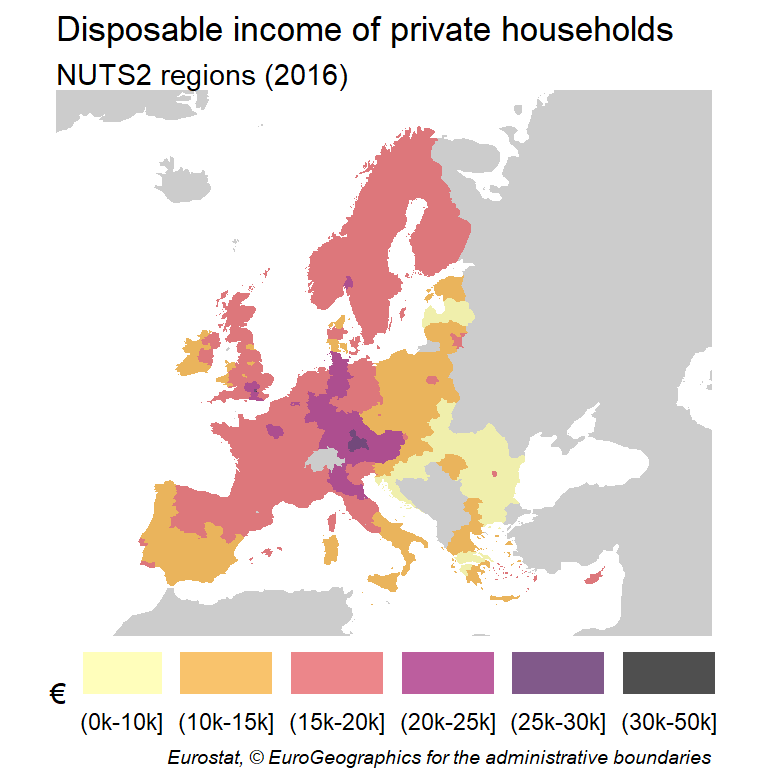# Choropleth maps in ggplot2

A choropleth map is a type of map where different geographic areas are colored based on a variable associated to each of those areas. A choropleth map provide an intuitive way to visualize how a specific variable (as population density, income, etc.) could vary across different geographic areas.

We would use the data included on the `giscoR` package, that provides map data on `sf` format and an example dataset `tgs00026`, that includes the disposable income of private households at NUTS2 level. On this example, we would use the year 2016 as a reference.

## Base map

In first place, we would need to get the spatial data that contains the geographical information to be used on the plot. On `giscoR`, we can select the NUTS geometries with `giscoR::gisco_get_nuts()` and visualize the object with `geom_sf`:

``````# install.packages("sf")
library(sf)
# install.packages("dplyr")
library(dplyr)
# install.packages("ggplot2")
library(ggplot2)
# install.packages("giscoR")
library(giscoR)

# Year
year_ref <- 2016

# Data
nuts2 <- gisco_get_nuts(year = year_ref,
resolution = 20,
nuts_level = 2) %>%
select(NUTS_ID, NAME_LATN)

# Base map
ggplot(nuts2) +
geom_sf()``````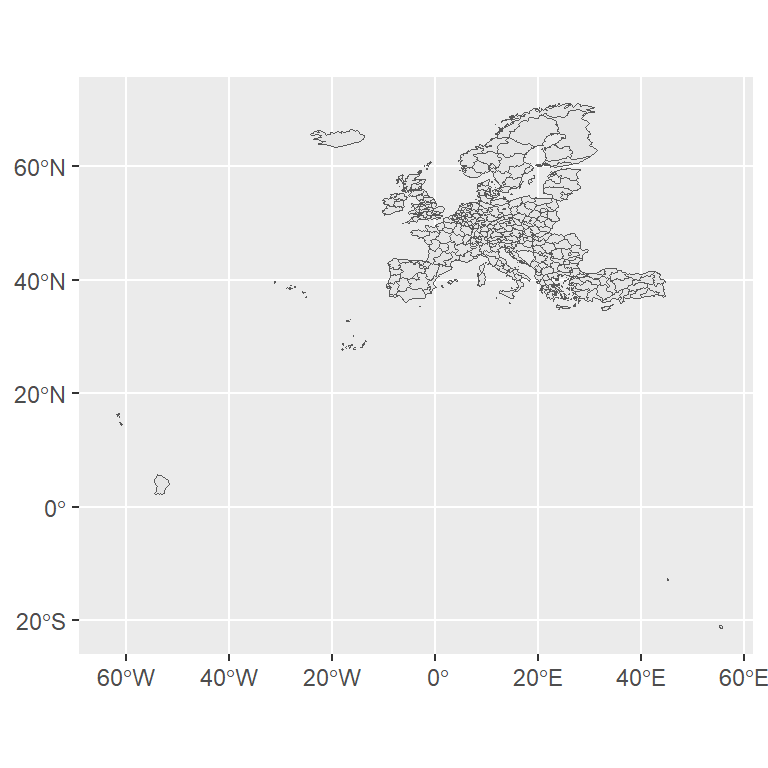## Limits and projection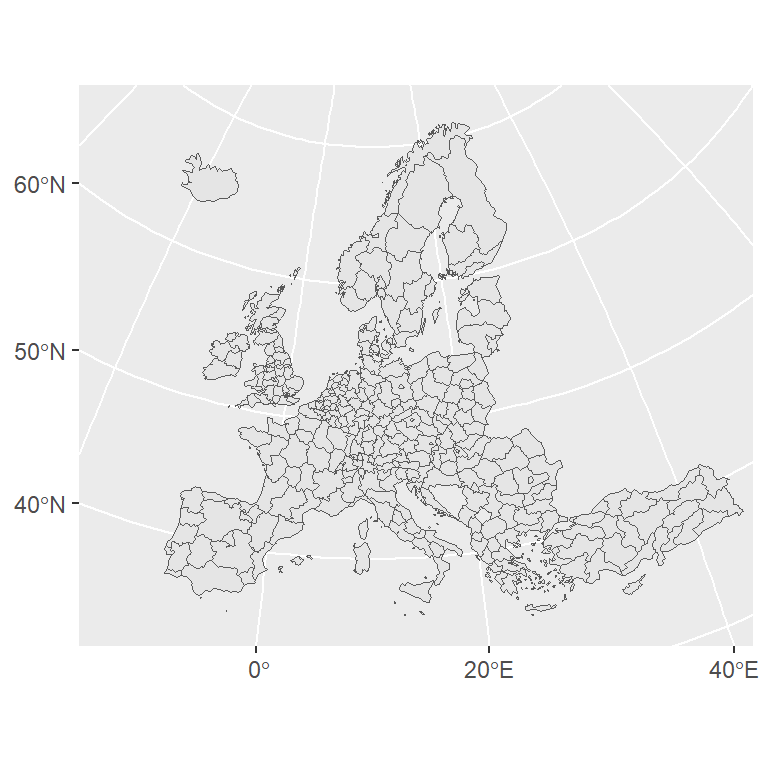We can improve the map by changing the Coordinate Reference System (CRS) to ETRS89-extended / LAEA Europe (EPSG code: 3035) and focusing the map on the continental area. We can use `xlim` and `ylim` to define the limits of the map:

``````# Transform the shape
nuts2_3035 <- st_transform(nuts2, 3035)

ggplot(nuts2_3035) +
geom_sf() +
xlim(c(2200000, 7150000)) +
ylim(c(1380000, 5500000))``````

## Join map and data

One of the advantages of `sf` is that the geographical objects behave as data frames, so we can perform a simple join to merge the statistical data with the geographical data.

``````# Filter to select data from 2016
disp_income <- giscoR::tgs00026 %>%
filter(time == year_ref) %>%
select(-time)

nuts2_3035_data <- nuts2_3035 %>%
left_join(disp_income, by = c("NUTS_ID" = "geo"))``````

Now, we would have an object with the data from `giscoR::tgs00026` and a `geometry` column, that includes the geographical data coordinates.

## Basic choropleth map

As a result of the previous process, we have available an `sf` object with the statistical data to be plotted. We can now proceed to create a basic choropleth map with `ggplot2`, passing the values (`values` column) of interest to the `fill` argument of `aes`: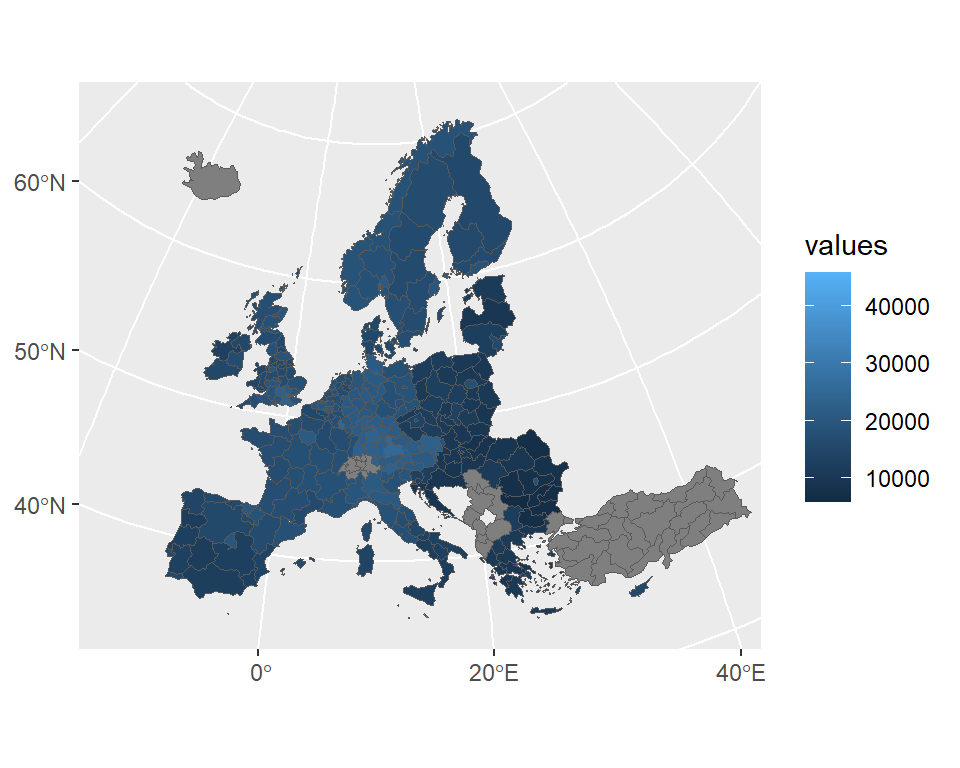Default colors

``````ggplot(nuts2_3035_data) +
geom_sf(aes(fill = values)) +
xlim(c(2200000, 7150000)) +
ylim(c(1380000, 5500000))``````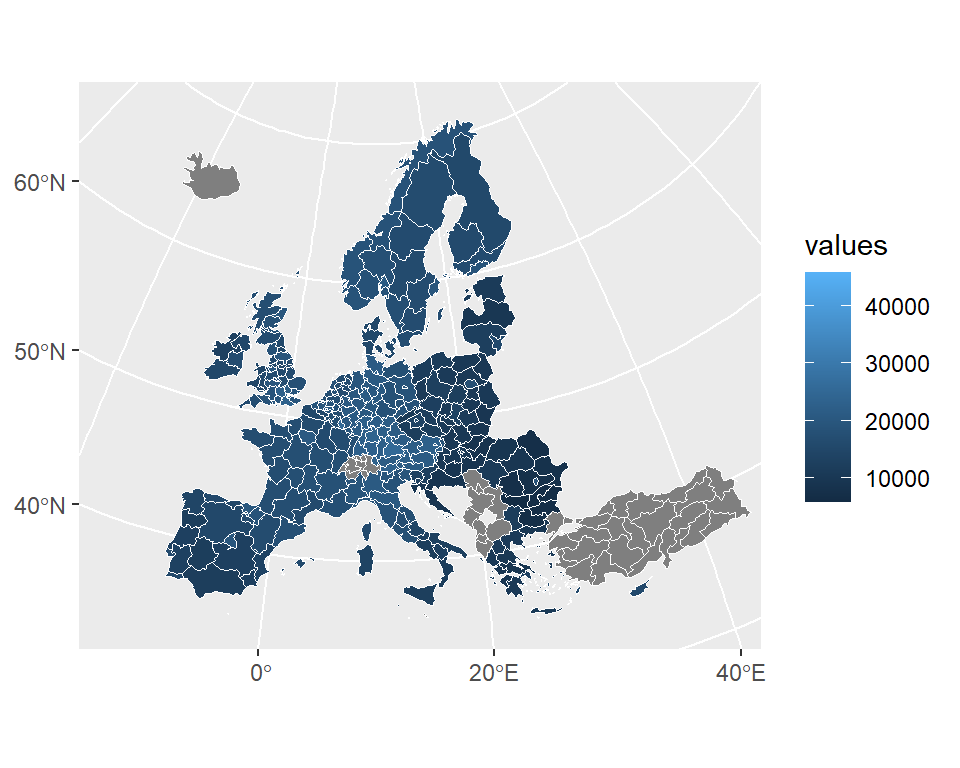Lines customization

Note that you can change the color, the type and the width of the lines with the corresponding arguments of the `geom_sf` function.

``````ggplot(nuts2_3035_data) +
geom_sf(aes(fill = values),
color = "white",
linetype = 1,
lwd = 0.25) +
xlim(c(2200000, 7150000)) +
ylim(c(1380000, 5500000))``````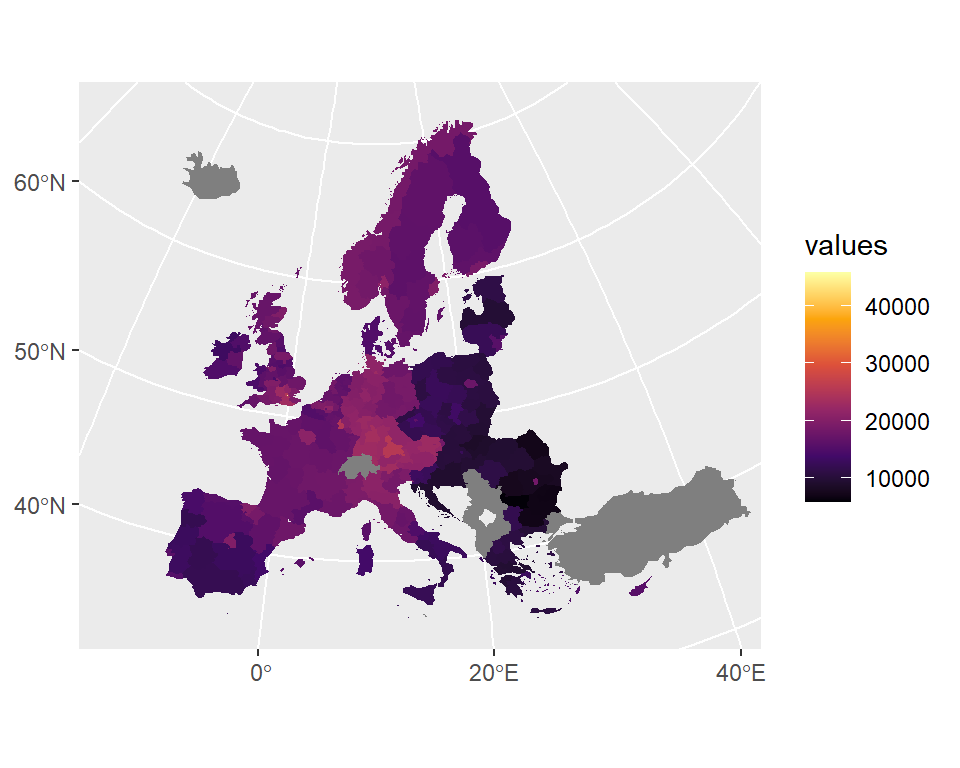Fill color customization

You can also specify a continuous color scale, such as `scale_fill_viridis_c`.

``````ggplot(nuts2_3035_data) +
geom_sf(aes(fill = values),
color = NA) +
xlim(c(2200000, 7150000)) +
ylim(c(1380000, 5500000)) +
scale_fill_viridis_c(option = "B")``````

We can still modify some features of the map to create a more eye-catching plot.

We are going to modify some features of the map in order to create a more advanced map. We would use custom breaks and colors to create a categorical map derived from the continuous disposable income data. Additionally, we would plot also surrounding countries not included in the sample.

We start by getting the whole countries of the world, that would be used as our base map.

``````# Get all countries and transform to the same CRS
cntries <- gisco_get_countries(year = year_ref,
resolution = 20) %>%
st_transform(3035)

# Plot
# First overlay with the whole world
geom_sf(data = cntries, fill = "grey80", color = NA) +
# Set limits
xlim(c(2200000, 7150000)) +
ylim(c(1380000, 5500000))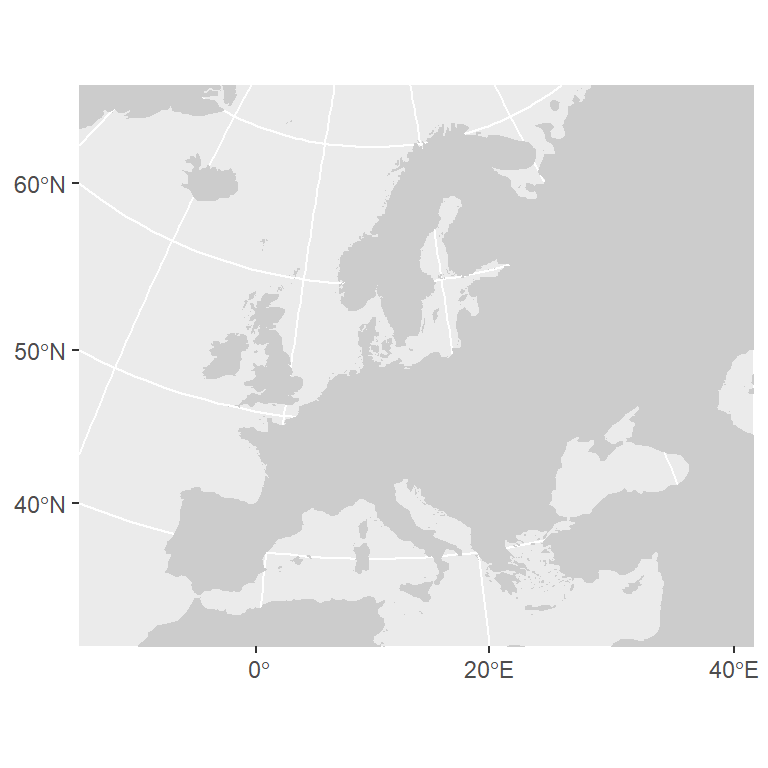Now, we can start preparing the choropleth overlay. We start by removing the data values with `NA` and creating discrete categories. We also prepare here the labels to be included on the legend of the map.

``````# Clean NAs from initial dataset
nuts2_3035_data_clean <- nuts2_3035_data %>%
filter(!is.na(values))

# Create breaks and discretize values
br <- c(0, 10, 15, 20, 25, 30, 50) * 1000

nuts2_3035_data_clean\$disp_income <- cut(nuts2_3035_data_clean\$values,
breaks = br,
dig.lab = 5)

# Create custom labels - e.g. (0k-10k]
labs <- c(0, 10, 15, 20, 25, 30, 50)
labs_plot <- paste0("(", labs[1:6], "k-", labs[2:7], "k]")``````

Finally, we choose the `Inferno` palette included on the `hcl.colors` function (R >= 3.6) and proceed to create the final map:

``````# Palette
pal <- hcl.colors(6, "Inferno", rev = TRUE, alpha = 0.7)

geom_sf(data = nuts2_3035_data_clean,
aes(fill = disp_income), color = NA) +
# Labs
labs(title = "Disposable income of private households",
subtitle = "NUTS2 regions (2016)",
fill = "€") +
# Custom palette
scale_fill_manual(values = pal,
drop = FALSE,
na.value = "grey80",
label = labs_plot,
# Legend
guide = guide_legend(direction = "horizontal",
nrow = 1,
label.position = "bottom")) +
# Theme
theme_void() +
theme(plot.caption = element_text(size = 7, face = "italic"),
legend.position = "bottom")``````